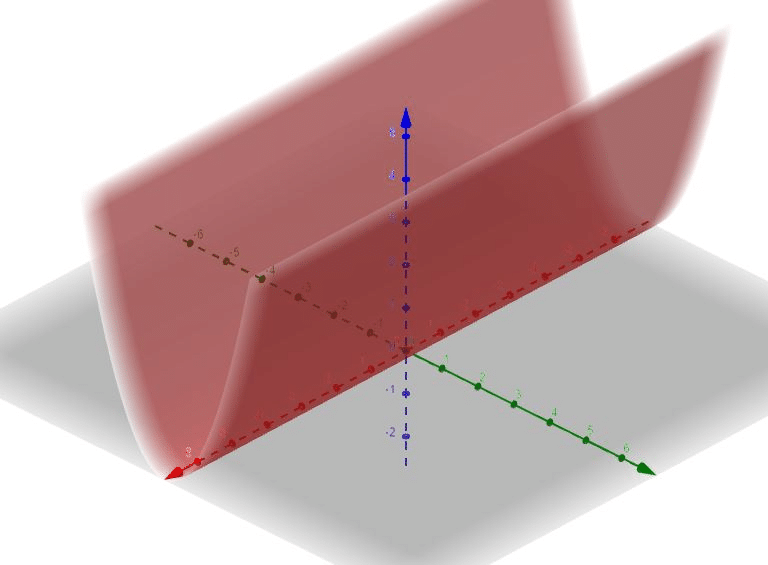# What Does The Equation Z = Y2 Represent?

We thoroughly check each answer to a question to provide you with the most correct answers. Found a mistake? Let us know about it through the REPORT button at the bottom of the page.

What does the equation z = y^2 represent?

## Explanation

Recall from the previous subquestion that we have identified y=x^2 as a parabola in R^2 in the xy-plane. Likewise, z=y^2 represents a parabola in R^2 in the yz-plane.

Extending the same logic in R^3 from the previous question, since the variable x is missing, the equation is also a parabolic cylinder in R^3.

This time, its trace is a parabola in the yz-plane with the parabola pointing up in the direction of the +z-axis and its rulings are parallel to the x-axis. See the attached image for reference.